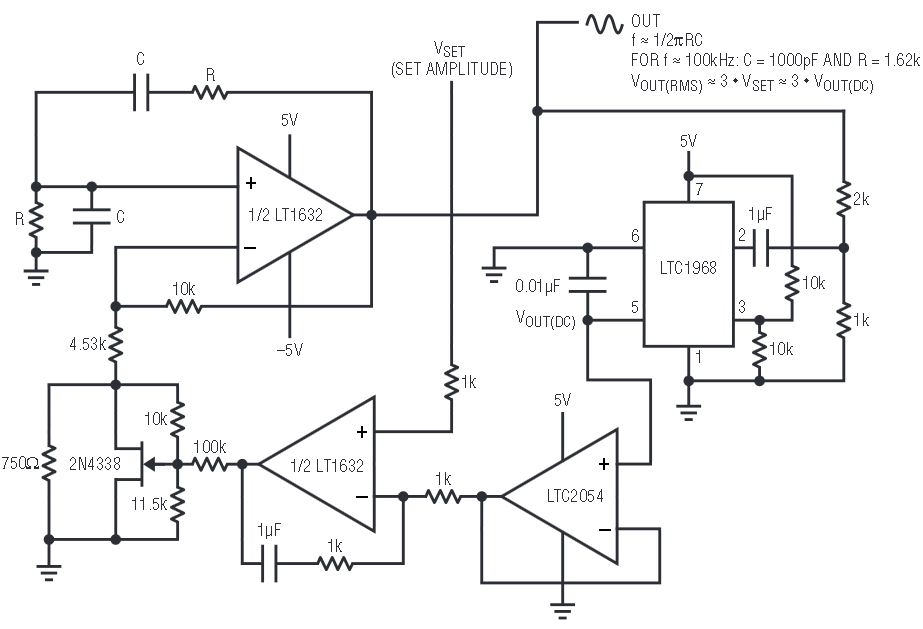# Low-Distortion Sine Wave Oscillator with Precise RMS Amplitude Stability

Many applications require a frequency and/or amplitude-stable sine wave as a reference for calibration or measurement. Low harmonic distortion is also required for meaningful results in applications such as LVDT signal conditioning, ADC testing, and, of course, harmonic distortion testing. Many sine wave generation techniques simply cannot achieve the low harmonic distortion and amplitude stability required of a precision sine wave reference. The technique shown here generates a sine wave with less than 0.003% distortion and 0.1% amplitude stability.

Figure 1 shows a simple oscillator circuit consisting of a Wien bridge oscillator core and an amplitude stabilization loop. The LT1632 high-speed low-distortion amplifier and its positive feedback RC network generate the oscillations. The amplitude, and amplitude stability, of the sine wave is controlled via a negative feedback loop comprising an LTC1968 RMS-to-DC converter, an LTC2054 buffer, and an LT1632 error amplifier.Figure 1. Schematic of the sine wave oscillator, consisting of a low-distortion LT1632-based Wien bridge oscillator core and an LTC1968-controlled amplitude stabilization loop. The output of the LTC1968 equals the RMS level of the sine wave divided by 3.

The oscillation occurs at a frequency of 1/(2πRC), where R and C are the positive feedback components of the amplifier. The attenuation of the negative feedback network is approximately 3, to match the attenuation encountered in the positive feedback network. The 2N4338 JFET acts as a variable resistor whose resistance changes according its the gate-source voltage bias. Changing the bias of the JFET adjusts the gain of the oscillator, and thus the amplitude of the resulting sine wave signal. The turn-on and amplitude settling time of this circuit are dominated by the settling time of the LTC1968, which is typically around 1 millisecond with a 0.01μF averaging capacitor.

The LTC1968 precisely measures the RMS amplitude of the LT1632’s output sine wave and gives a DC output that corresponds to the RMS level of the sine wave divided by three. The resistive attenuator at its input allows the LTC1968 output to remain within its low-error region of ≤ 1V for up to 3VRMS output sine waves.

The LTC2054 buffers the output of the LTC1968 for minimal error due to output loading, and the LT1632 error amplifier compares the RMS level of the sine wave with VSET, which sets the desired RMS amplitude. The error amp controls the gate-source voltage bias of the JFET to modulate the amplitude accordingly. As shown, the output amplitude of the sine wave is:

The 10k-11.5k resistive attenuator at the gate of the JFET compensates for the channel modulation effects of the JFET, which otherwise would cause severe harmonic distortion in the circuit.

As measured with a Hewlett-Packard 3589A Spectrum Analyzer, the harmonic distortion of this circuit with a 100kHz, 1VRMS sine wave output is –92dBc (0.0025%). The amplitude stability is better than –60dBc (0.1%). With a 2VRMS output, the circuit yields only slightly degraded performance, at –80dBc (0.01%) harmonic distortion and –55dBc (0.18%) amplitude stability.

The LTC1968 can measure the amplitude of sine waves up to 500kHz in frequency with less than 1% absolute error (independent of the amplitude stability of the circuit). Producing higher frequency sine waves using this circuit is possible, up to the 15MHz bandwidth of the LTC1968.